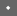USENIX Technical Program - Paper - Smartcard 99    [Technical Program]

## Investigations of Power Analysis Attacks on Smartcards

Motorola Labs, Motorola
tomas@ccrl.mot.com

Ezzy A. Dabbish

dabbish@ccrl.mot.com

Robert H. Sloan1
Dept. of EE and Computer Science, University of Illinois at Chicago
sloan@eecs.uic.edu

#### Abstract

This paper presents actual results from monitoring smartcard power signals and introduces techniques that help maximize such side-channel information. Adversaries will obviously choose attacks that maximize side-channel information, so it is very important that the strongest attacks be considered when designing defensive strategies. In this paper, power analysis techniques used to attack DES are reviewed and analyzed. The noise characteristics of the power signals are examined and an approach to model the signal to noise ratio is proposed. Test results from monitoring power signals are provided. Next, approaches to maximize the information content of the power signals are developed and tested. These results provide guidance for designing smartcard solutions that are secure against power analysis attacks.

### Introduction

Cryptographers have traditionally analyzed cipher systems by modeling cryptographic algorithms as ideal mathematical objects. Conventional techniques such as differential  and linear  cryptanalysis are very useful for exploring weaknesses in algorithms represented as mathematical objects. These techniques, however, cannot address weaknesses in cryptographic algorithms that are due to a particular implementation in hardware. The realities of a physical implementation can be extremely difficult to control and often result in the leakage of side-channel information. Techniques developed in  show how surprisingly little side-channel information is required to break some common ciphers. Attacks have been proposed that use such information as timing measurements [4 ,5 ], power consumption , electromagnetic emissions  and faulty hardware [8 ,9 ]. Eliminating side-channel information or preventing it from being used to attack a secure system is an active area of research.

A growing number of researchers are beginning to address this issue of implementation. Systems that rely on smartcards to provide security are of particular concern. In such systems, smartcards are often viewed as tamper-resistant devices that are secure against all but the most determined and well-financed attackers. However, this reliance on the tamper resistance of smartcards needs to be carefully scrutinized  . This becomes even more important in light of the recent attacks using side-channel information. It is often the case that important data stored on smartcards, such as a cryptographic key or an authentication certificate, needs to be kept secret to prevent counterfeiting of cards or the breaking of a system's security. Examples of smartcard systems that rely on the secrecy of the data resident on smartcards can be found in  . Such systems are potentially vulnerable because every time the smartcard system performs a computation using the secret data, side-channel information may be leaked.

Of all the previously mentioned sources of side-channel information, power measurements are perhaps the most difficult to control. Ultimately all calculations performed by a smartcard operate on logical ones or zeros. Current technological constraints result in different power consumptions when manipulating a logical one compared to manipulating a logical zero. An attacker of a smartcard can monitor such power differences and obtain useful side-channel information. Differential Power Analysis (DPA)  is a statistical approach to monitoring such power signals from a smartcard. Kocher et al.  claim one can monitor the actions of a single transistor within a smartcard using DPA. In  , the authors outline a specific DPA attack against smartcards running the DES  algorithm.

The purpose of this paper is to present actual results from monitoring smartcard power signals and to introduce techniques that help maximize such side-channel information. Whereas  showed how little side-channel information is required by an attacker, this paper takes the alternate approach and provides a first step towards showing how such information can be maximized. Adversaries will obviously choose attacks that maximize side-channel information, so it is very important that the strongest attacks be considered when designing defensive strategies. In this paper, power analysis techniques for attacking DES are reviewed and analyzed. The noise characteristics of the power signals are examined and an approach to model the signal to noise ratio is proposed. Test results from monitoring power signals are provided. Next, approaches to maximize the information content of the power signals are developed and tested. These results provide guidance for designing smartcard solutions that are secure against power analysis attacks.

### Smartcard Power Dissipation

The circuit shown in Figure 1 gives a simple lumped component model that is useful for understanding power dissipation measurements. Power dissipated by the smartcard can be monitored at the ground pin of the smartcard by using a small resistor (R1) in series between the VSS pin on the card and the true ground. Current moving through R1 creates a time varying voltage that can be sampled by a digital oscilloscope. The current flows out of the smartcard through a bond wire that acts as an inductor Lbond. The values of the inductor, Lbond, and the capacitors will determine the shape of the power signal that is observed at Vscope. In a CMOS circuit, most power is dissipated when the circuit is clocked. This is known as dynamic power dissipation  . As Vgate changes from 0 to 5 volts, the transistors Q1 and Q2 are both conducting for a brief period causing current to flow from Vdd to ground. Also during this time, the capacitor Cload will be discharged (or charged) causing more (or less) current to flow through the VSS pin.Information useful to a cryptanalyst is leaked because the amount of current being drawn when the circuit is clocked is directly related to the change of state of Cload or the resulting current drawn by the other gates attached to Cload. On a microprocessor, each clock pulse causes many bit transitions to occur simultaneously. In a typical smartcard microprocessor, a large portion of the power dissipation occurs in the gates attached to internal buses. Our experiments showed that activity on the data and address bus is a dominant cause of power consumption changes. These changes can be observed at Vscope.

Two types of information leakage from the data bus that have been observed are Hamming weight leakage and transition count leakage. Hamming weight information leaks when the dominant source of current is caused by the discharging of Cload. A situation where Hamming weight information leaks is when a precharged bus design is used. In this case, the number of zeros driven onto the precharged bus directly determines the amount of current that is being discharged. Transition count information leaks when the dominant source of current is due to the switching of the gates that are driven by the data bus. When the data bus changes state, many of the gates driven by the bus will briefly conduct current. Thus, the more bits that change state, the more power that is dissipated.Experimental results that show transition count leakage from a typical 8-bit, microprocessor-based smartcard are given in Figure 2 . In this figure, an 8-bit data byte from memory is transferred into a register. Initially, the bus contains the memory address, but after a clock pulse, the data from memory is put onto the bus. The magnitude of the voltage pulse is directly proportional to the number of bits that changed. Waveforms for different values of memory data are plotted on top of each other to effectively show this relationship. The difference in voltage between i transitions and i+1 transitions is about 6.5 mV. We observed similar plots for smartcards that leak Hamming weight information. The type of information that a particular smartcard leaks depends on the circuit design of the microprocessor and the type of operation being performed by the card. For instance, accessing EEPROM may yield different information than accessing RAM. Knowing which type of information is leaked will enable an adversary to optimize an attack strategy.

### Simple Power Analysis (SPA)

An SPA attack, as described in , involves directly observing a system's power consumption. Different attacks are possible depending on the capabilities of the attacker. In some situations the attacker may be allowed to run only a single encryption or decryption operation. Other attackers may have unlimited access to the card. The most powerful attackers not only have unlimited access, but also have detailed knowledge of the software and hardware running in the card. If an attacker can determine where certain instructions are being executed, it can be relatively simple to extract useful information. For example, during the PC1 permutation in DES, we could determine the Hamming weight of each key byte by measuring the pulse height at the cycle of the instruction that accesses this data. In an 8-bit microprocessor, knowing the Hamming weight of all eight DES key bytes reduces the brute-force search space from 256 to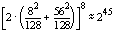orkeys depending on whether or not the parity bits are used. This was just an example; with algorithms using more key bits than DES or with triple-DES, knowing Hamming weight information alone does not help much with this type of brute-force attack.

A more powerful attack can result if the attacker can see Hamming weight information about the key bytes and also information about shifted versions of the key bytes. In DES, such information can be leaked when shifting the C and D registers. In fact, given the weight of each byte for eight of the C and D shifts there is enough information to solve for the value of every key bit using the equation

######(1)
whereis a 56x1  vector of Hamming weights, wiis a  56x1 binary vector of the key bits, kj; and A is a 56x56 binary matrix such that Aij is 1 if and only if weight wi includes key bit kj. Even algorithms with more than 56 key bits, such as triple-DES would be vulnerable to this attack.

If transition count information rather than Hamming weight information is available, an SPA attack can still be mounted, but it may be more difficult. The attacker would need to know the contents of the data bus before or after the data being sought is accessed. Many times this data is easy to determine because it is a fixed address or an instruction opcode. Attackers with access to the code can easily get this data and set up equations similar to Equation (1) . Other less knowledgeable attackers may need to resort to trial and error to determine the correct equations. Due to the limited number of possibilities such an approach is reasonable.

Our experiments confirmed that poor implementations of DES will almost always be vulnerable to SPA attacks. Shifting the key bytes or the use of conditional branches to test bit values can be especially vulnerable. Also, if the code or portions of the code run in variable time, power analysis could be used to enable a timing attack  . Fortunately, implementors of cryptographic algorithms have known about these issues for a number of years. Kocher et al.  reported that it is not particularly difficult to build SPA-resistant devices. However, the attackers keep getting smarter so further research and vigilance will always be necessary.

### Differential Power Analysis (DPA)

A DPA attack, described in  and reviewed here, is more powerful than an SPA attack because the attacker does not need to know as many details about how the algorithm was implemented. The technique also gains strength by using statistical analysis to help recover side-channel information. The objective of the DPA attacks described in this paper is to determine the secret key used by a smartcard running the DES algorithm. These techniques can also be generalized to attack other similar cryptographic algorithms.

A DPA attack begins by running the encryption algorithm for N random values of plain-text input. For each of the N plain-text inputs, PTIi, a discrete time power signal, Sij, is collected and the corresponding cipher-text output, CTOi, may also be collected. The power signal Sij is a sampled version of the power consumed during the portion of the algorithm that is being attacked. The i index corresponds to the PTIi that produced the signal and the j index corresponds to the time of the sample. The Sij are split into two sets using a partitioning function, D( . , . , . ):
######(2)

The next step is to compute the average power signal for each set:
######(3)

where |S0| + |S1| = N. By subtracting the two averages a discrete time DPA bias signal, T[j], is obtained:
######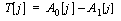(4)

Selecting an appropriate D function will result in a DPA bias signal that an attacker can use to verify guesses of the secret key. An example of such a D function is as follows:
######(5)
where
C1 = the 1 bit of CTOi that is XOR'ed with bit #1 of S-box #1

C6 = the 6 bits of CTOi that are XOR'ed with subkey K16

K16 = 6 bits of the 16th round subkey feeding into S-box #1

SBOX1(x) = a function returning bit #1 resulting from looking up x in S-box #1

The D function of Equation (5) is chosen because at some point during a DES implementation, the software needs to compute the value of this bit. When this occurs or anytime data containing this bit is manipulated, there will be a slight difference in the amount of power dissipated depending on whether this bit is a zero or a one. If this difference is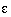, and the instructions manipulating the D bit occurs at times j*, the following expected difference in power equation results:

######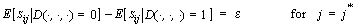(6)
When j is not equal to j*, the smartcard is manipulating bits other than the D bit, and the power dissipation is independent of the D bit:
######(7)
As the number N of PTI inputs is increased, Equation (4) , converges to the expectation equation:
######(8)
Thus, a review of Equation (6) , (7) and (8) shows that if enough PTI samples are used, T[j] will show power biases ofat times j*, and will converge to zero all other times. Due to small statistical biases in the S-box outputs, the assumption of independence in Equation (7) is not completely true. In reality, T[j] will not always converge to zero; however the largest biases will occur at times j*.

One input to the D function was K16, six bits of the subkey. The attacker does not know these bits, but can use brute force and try all 26 possible values. For each guess, the attacker constructs a new partition for the power signatures and gets a new bias signal, T[j]. If the proper D function was chosen, the bias signal will show spikes whenever the D bit was manipulated. If the D function was not chosen correctly (i.e., the wrong subkey bits were guessed), then the resulting T[j] will not show any biases. Using this approach, an attacker can determine the six subkey inputs to S-box #1 at round 16 of DES. Repeating this approach for the seven other DES S-boxes allows the attacker to learn the entire round 16 subkey (48 bits). The remaining 8 bits can be found by brute force or by successively applying this approach backwards to previous rounds. Figure 3 compares the bias signal for a correctly chosen key to that of an incorrectly chosen key. The signal for the incorrect key shows a few small biases, but the correct key has biases that are twice as large, so it is easily recognized. We created the signals in Figure 3 using N = 1300.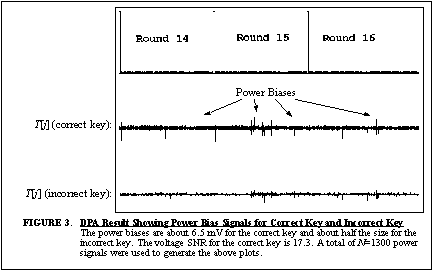### Noise in Power Analysis Attacks

There is a rich history of methods to reduce noise in electrical circuits. Many good textbooks have been written on this topic (e.g.,  ). Techniques for noise reduction are particularly important when trying to measure side-channel emanations because the magnitude of these signals can be extremely small. The DPA attack uses averaging to reduce noise, but it is important to investigate other strategies that may lead to further reductions in the amount of noise. Experts quoted in  report that one way to prevent a power analysis attack is to mask the side-channel information with random calculations that increase the measurement noise. A good understanding of the achievable noise levels using typical electronic measuring techniques is needed to evaluate the effectiveness of such a solution.

### Noise Characteristics

There are four types of noise present when performing power analysis of smartcards: external, intrinsic, quantization and algorithmic. External noise is generated by an external source and coupled into the smartcard. Intrinsic noise is due to the random movement of charge carriers within conductors. Quantization noise is due to the quantizer in the A/D that is used to sample the power signals. Algorithmic noise is due to the randomness of the data bytes being processed by the smartcard. Intrinsic and quantization noise are small when compared to present day side-channel signals, but will likely play a larger role as future designs minimize side-channel signals. External noise can be reduced through careful use of measuring equipment, good circuit design practices and filtering. Algorithmic noise can be reduced if an attack strategy can use unbiased random data that can be averaged out.Figure 4 illustrates a sample of the noise measured in a power analysis experiment. The top waveform, Nj, was determined using the following equation:
######where the value of E[Sj] was estimated by averaging the power traces obtained when encrypting the same input data 5,000 times and Sj is one of the traces. Clearly any deviation of Nj from zero should be considered noise. The noise shown in Figure 4 has a slow beat frequency around 182 Khz that could be due to external coupling from test equipment or more likely due to the superposition of the digital oscilloscope's sampling frequency and the smartcard's clock frequency. The power spectrum of the noise also reveals significant spikes at the smartcard's clock frequency and its harmonics. A close-up of the noise reveals that a significant amount of the noise energy is concentrated at the edges of the smartcard's 4 Mhz clock edges.
Figure 4 does not contain algorithmic noise since the data being encrypted was kept constant. The effect of algorithmic noise would be to cause spikes to appear in the portion of the signal where the data is being processed. These spikes could be used by an attacker to mark locations in the power trace that are data dependent. Algorithm noise can be modeled as shot noise using a generalized Poisson random process  :
######In this case, ci is a random variable (r.v.) corresponding to the Hamming weight of the data being processed, ji is a Poisson r.v. that models the seemingly random locations of the data dependencies and F would be the shape of the bias signal. If random data is used, then ci would have a binomial distribution. An accurate model of the various noise components would be very useful for simulating the effectiveness of DPA attacks and solutions.

### Filtering the Noise

Filtering strategies can be used to reduce the noise shown in Figure 4 , but care needs to be taken so that the components necessary to create a power bias signal are not affected. The algorithmic noise can be reduced by averaging using unbiased random data. Filtering will not work on algorithmic noise because any filter would also reduce the desired bias signal. If the noise in Figure 4 is assumed to be white noise, then the optimal filter that maximizes the signal-to-noise ratio (SNR) is the matched filter. We designed and tested such a filter for the bias signal shown in Figure 3 . The original voltage SNR was 17.3. After using the matched filter, the SNR was increased to 23.2. This gives some indication of how much an attacker with a perfect matched filter can improve the SNR.

### The Effect of Averaging

A DPA attack is successful because averaging reduces noise energy, thus revealing any concentrations of signal energy that occur at constant positions in time. To see the effect of averaging on the noise refer back to Equation (3) . Let the average variance of Sij be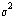and assume Sij is independent of Skj when. If the N signals were equally split between sets S0 and S1, then the variance of A0 and A1 is. Therefore, when A0 and A1 are combined the resulting DPA bias signal has a variance of.

The averaging effect on the signal was shown in Equation (6) produce a bias spike of size. The D function, proposed in Equation (5) , has the effect of fixing one bit of the data being processed. If a data word is m bits wide, then the remaining unfixed bits in the word have an average Hamming weight of (m-1)/2 with a variance (i.e., algorithmic noise) of (m-1)/4. Averaging over N samples has a similar effect on this signal variance as it did on the noise variance. Assuming independence of the different sources of noise yields the following signal and noise parameters:
######(9)

In the variance of the noise, the term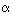is the percentage of T[j] that is dependent on the data being encrypted, thus the percentage of algorithmic noise. The success of a DPA attack depends on distinguishing the signal from noise, so the final voltage SNR is:
######(10)

Equation (10) can be checked with previously measured experimental results. Setting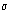=7.5 mV (from Figure 4 ),= 6.5 mV (from Figure 2 ), m = 8, N = 1000, andyields SNR = 7.5. Experimental results using these parameters showed a SNR between 7 and 10, thus confirming that Equation (10) is a valid approximation.

### Maximizing DPA Bias Signal

One way to increase the SNR in Equation (10) is to increase. The value ofis dependent on the number of bits output by the D function. In Equation (5) , D outputs only one bit, but in general the D function could output d bits. In this general case= dl, where l is a constant equal to the voltage difference seen between two data words with Hamming weight i and i+1. Figure 2 showed that these differences can be considered approximately equal, for all i.

When performing the differential power analysis there would be three sets used for partitioning:
######The other DPA equations would all remain the same and the power signals in set S2 would not be used. The following signal and noise equations would result:

### 4-bit DPA Attack on DES

In DES, a 4-bit D function based on the four bits output from an S-box can be used to partition the sets S0 and S1. Equation (5) can be modified to output four bits:
######(12)

where SBOX14(x) returns all four bits resulting from looking up x in SBOX #1. Similar D functions would be used for the other S-boxes to enable all the key bits to be eventually determined.

The question arises as to whether Equation (12) will result in partitions that are unbiased enough so that the incorrect choices of K16 will result in random power signals that average to zero. A simulation was written to see what type of biases could be expected. The results for 4-bit DPA and 1-bit DPA using random PTI inputs and a typical key are graphed in Figure 5 . The graph shows that the expected Hamming weight biases of the S-box #1 lookup for each of the 64 possible key guesses. The correct key guess clearly shows the most bias; however in the case of 4-bit DPA there is a case where an incorrect key guess has the same magnitude bias as the correct key. In this case, if the attacker did not know the sign of the expected bias, a very small brute-force search might be needed.### Multiple Bit DPA

In general, other multiple bit D functions can be defined. In software implementations, the S-box lookups are performed using a load instruction from a table storing all the S-box data. To compress the table size, the four bit S-box data is frequently stored in pairs of two or more, depending on the word size of the processor. If the attacker knows how the S-box data is packed into this table, it would be possible to maximize the bit biases even further. For example, power signals with S-box lookups that yield many zeros can be separated from signals with S-box lookups containing many ones. Again, the number of bits that can be biased will proportionally increase the SNR.
Another way to mount an attack would be to partition S0 and S1 based on the addresses used for the S-box lookups rather than the data. The attacker forms two partitions, one that maximizes the number of address bus transitions and one that minimizes the number of address bus transitions. Power signatures from both partitions are averaged and then subtracted. If the address bus is larger than the data bus, even larger biases could be achieved.

When mounting a d-bit DPA attack the attacker may need to use more power signals. After all, an average of 1-21-d of the power signals are placed in partition S2 and are unused. This suggests that an attacker cannot increase d too much without requiring an excessive number of power signals. An attacker running 1-bit DPA using N signals would need Nd = 2d-1N/d2 power signals to maintain the same SNR when running d-bit DPA. Table 1 shows that for small values of d actually fewer power signals are necessary and with 8-bit DPA only twice as many signals are needed. The main advantage of d-bit DPA is that the resulting signal levels are magnified d times.
Experiments were run on a smartcard to confirm the effectiveness of 4-bit, 8-bit and address-bit DPA. The resulting signal levels are shown in Table2. In these experiments, the 8-bit DPA attack was only able to create an average bias of 6.0 bits because the S-box data was never all zeros or all ones. The address-bit DPA had similar problems so only an average bias of 6.5 bits was achieved. As can be seen the signal levels for these multiple bit DPA attacks are much stronger. It is clear that countermeasures to DPA attacks need to consider all these more powerful attacks.

Table1:
 d 1 2 3 4 5 6 7 8 N N 0.5N 0.44N 0.5N 0.64N 0.88N 1.3N 2N

Table 2:
 Attack type: 1-bit DPA 4-bit DPA 8-bit DPA Address DPA Signal Level: 9.3 mV 38.5mV 79.5mV 74.4mV

### Hiding the DPA Bias Spike

One suggested solution to prevent DPA attacks is to add random calculations that increase the noise level enough to make the DPA bias spikes undetectable. The results presented in this paper give some indication of how much noise needs to be added. The main goal is to add enough random noise to stop an attack, but to add minimal overhead. A rough approximation of the noise necessary can be found by looking at the probability density function (p.d.f.) of the DPA bias signal plotted in Figure 6 . The DPA spike obviously stands out from the noise because it is very improbable that it was generated by noise. Ideally, the p.d.f. of the bias signal would stretch out to cover the DPA spike or the DPA spike would be reduced so that it is closer to the noise. One suggested design criterion is that the DPA bias spike have equal amounts of noise distributed above and below it. This means the median of the noise signal would be at the level of the DPA bias spike. If the noise were Guassian, the median would be when the SNR=0.67. One could then use Equation (11) to determine what amount of noise is necessary to prevent the desired level of attack. Of course a different strategy would need to be used if the noise p.d.f. was not normal or the noise around the DPA spike was non-stationary. The fact that the DPA bias spikes and most of the noise energy occurs near the clock edges can also be considered. Less noise may be necessary because the DPA spikes occur in these areas of high noise energy.### Future Work

Future research in this area will investigate power analysis attacks on hardware encryption devices and public-key cryptosystems. Preliminary work suggests that such systems will also be vulnerable, but specific attacks have not yet been evaluated. The analysis of more symmetric-key algorithms is also an important topic that will be investigated. Ways to design and implement new algorithms that are not vulnerable to these attacks will be researched. The solutions to prevent these types of side-channel attacks need to be carefully scrutinized. Comprehensive analysis techniques, testing procedures and more advanced modeling methods will be developed.

### Conclusions

Attacks that monitor side-channel information and in particular power analysis attacks have recently been getting much attention by experts in the smartcard industry. The results presented in this paper confirm that power analysis attacks can be quite powerful and need to be addressed. Ways an attacker might maximize the side-channel signals have been investigated and were found to be very effective. Solutions to prevent DPA attacks need to consider these advanced attacks in order to provide the maximum amount of security. Understanding the noise characteristics of the power signals is also very important. The experimental and theoretical results presented in this paper hopefully will be helpful for modeling the problem and designing the solutions.

Much of the concern in industry is how to safeguard existing products. These products are mostly software implementations similar to the ones examined in this paper. The new attacks and analysis results presented in this paper are applicable to these designs and to future designs and hardware implementations. When creating a new hardware encryption circuit or algorithm implementation, a designer needs to ask the question: "What is the strongest potential power analysis attack that can be mounted by an attacker?" With the answer to this in mind, a designer can successfully protect against power analysis attacks.

### References

 E. Biham and A. Shamir, "Differential Cryptanalysis of DES-like Crytptosystems," Journal of Cryptography, Vol. 4, No. 1, 1991, pp. 3-72.

 M. Matsui, "Linear Cryptanalysis Method for DES Cipher," in Proceedings of Advances in Cryptology-Eurocrypt `93, Springer-Verlag, 1994, pp. 386-397.

 J. Kelsey, B. Schneier, D. Wagner, and C. Hall, "Side Channel Cryptanalysis of Product Ciphers," in Proceedings of ESORICS '98, Springer-Verlag, September 1998, pp. 97-110.

 P. Kocher, "Timing Attacks on Implementations of Diffie-Hellman, RSA, DSS, and Other Systems," in Proceedings of Advances in Cryptology-CRYPTO `96, Springer-Verlag, 1996, pp. 104-113.

 J. F. Dhem, F. Koeune, P. A. Leroux, P. Mestré, J. J. Quisquater and J. L. Willems, "A Practical Implementation of the Timing Attack," in Proceedings of CARDIS 1998, September 1998.

 P. Kocher, J. Jaffe, and B. Jun, "Introduction to Differential Power Analysis and Related Attacks," https://www.cryptography.com/dpa/technical, 1998.

 W. van Eck, "Electromagnetic Radiation from Video Display Units: An Eavesdropping Risk," Computers and Security, v. 4, 1985, pp. 269-286.

 D. Boneh and R. A. Demillo and R. J. Lipton, "On the Importance of Checking Cryptographic Protocols for Faults," in Proceedings of Advances in Cryptology-Eurocrypt `97, Springer-Verlag, 1997, pp. 37-51.

 E. Biham and A. Shamir, "Differential Fault Analysis of Secret Key Cryptosystems," in Proceedings of Advances in Cryptology-CRYPTO `97, Springer-Verlag, 1997, pp. 513-525.

 R. Anderson and M. Kuhn, "Tamper Resistance-A Cautionary Note," The Second USENIX Workshop on Electronic Commerce Proceedings, 1996, pp. 1-11.
 Cardtech/Securtech `98 - Conference Proceedings, Volume II: Applications, 1998.

 ANSI X.392, "American National Standard for Data Encryption Algorithm (DEA)," American Standards Institute, 1981.

 N. Weste and K. Eshraghian, Principles of CMOS VLSI Design, Addison-Wesley Publishing Company, 1993.

 E. R. Davies, Electronics Noise and Signal Recovery, San Diego, CA: Academic Press Inc., 1993.

 P. Wayner, "Code Breaker Cracks Smart Cards' Digital Safe," New York Times, June 22, 1998, pp. C1. (or https://www.nytimes.com/library/tech/98/06/biztech/articles/22card.html).

 A. Papoulis, Probability, Random Variables, and Stochastic Processes, New York: McGraw-Hill, Inc. 1991.

###### 1.Partially supported by NSF Grant CCR-9800070.

 This paper was originally published in the Proceedings of the 1st Workshop on Smartcard Technology, May 10-11, 1999, McCormick Place South Chicago, Illinois, USA Last changed: 18 Mar 2002 ml